Arithmetic Operations

Arithmetic operations can be thought of as manipulation of pixel values using additions, subtractions, multiplications, and divisions. They are also called linear point operations, since the output image value is a linear function of the input image value :

where DOUT is the output image value and DIN is the input pixel value.
Adding or subtracting a constant to and from a pixel value changes the brightness of an image. That is, the output image appears brighter when b > 0, and the image appears darker when b < 0. Figure 1.1 shows the effect of adding 50 to and subtracting 50 from every pixel value of the image BIRDGIRL. Multiplying or dividing the pixel values by a constant has the effect of increasing or decreasing the image's contrast. Figure 1.2 shows the effect of multiplying pixel values by 1.5 and 0.8. Arithmetic operations do create problems when the resulting pixel values are above 255 or below 0. Thus, clamping is usually used to set negative values to 0 and values above 255 to 255.
Listing 1.1 (arithmet) is a MATLAB implementation of the arithmetic operation function. The arithmetic operation function is implemented using table-lookup. Figure 1.1 shows the operation of a "DOUT = 3*DIN" arithmetic operation using table-lookup. Note that the pixel value 255 is also converted to 255 because of clamping.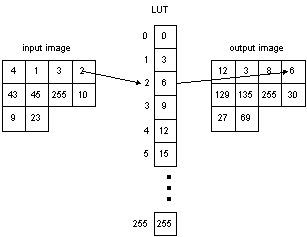Figure 1.1 An example of arithmetic operation using table-lookup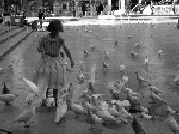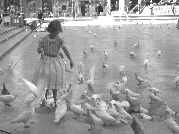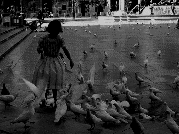a. Original BIRDGIRL b. Brightness increased by 50 c. Brightness decreased by 50

Figure 1.2 Arithmetic Operations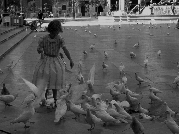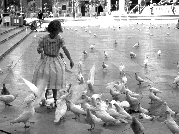a. Contrast decreased by a factor of 0.8 b. Contrast increased by a factor of 1.5

Figure 1.3 Arithmetic Operations

NAME
arithlut - performs an arithlutic operation on every input pixel.
SYNOPSIS
arithlut(input_file_name, output_file_name, a, b)
arithlut(input_file_name, output_file_name, [a,b])
DESCRIPTION
arithlut reads an image from the input file, performs the linear function "aX+b" for every pixel value, and writes the resulting image into the specified output file. The input image can be in any graphics format recognized by MATLAB. The graphics formats recognized by MATLAB include Windows Bitmap (bmp), Hierarchical Data Format (HDF), Joint Photographic Experts Group (JPEG), Windows Paintbrush (PCX), Tagged Image File Format (TIFF), Window Dump (XWD). The output image file is a TIFF file
 a --contrast scaling factor, reduced when a < 1 and increased when a > 1 b brightness scaling factor, reduced when b < 0 and increased when b > 0

EXAMPLES
arithlut('BIRDGIRL.TIF', 'ar_bg_1_50.tif', 1, 50)
The above example increases the brightness of BIRDGIRL by 50 (Figure 1.2).
arithlut('BIRDGIRL.TIF', 'ar_bg_1_n50.tif', 1, -50)
The above example decreases the brightness of BIRDGIRL by 50 (Figure 1.2)
arithlut('BIRDGIRL.TIF', 'ar_bg_08_0.tif', 0.8, 0)
The above example decreases the contrast of BIRDGIRL by multiplying every pixel by 0.8 (Figure 1.3).
arithlut('BIRDGIRL.TIF', 'ar_bg_15_0.tif', 1.5, 0)
The above example increases the contrast of BIRDGIRL by multiplying every pixel by 1.5 (Figure 1.3).